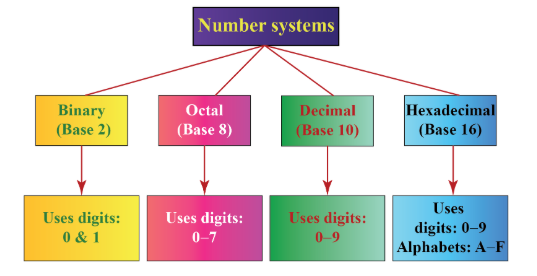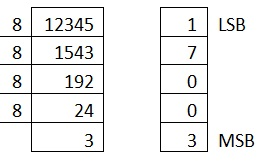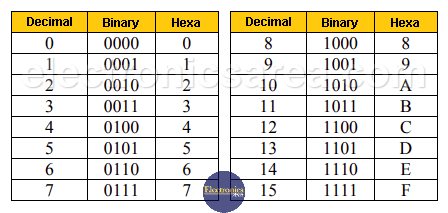# NUMBER SYSTEMS

Digital quantities can only take on discrete values while analog quantities vary over a continuous range of values. Examples of analog quantities include temperature, speed. A digital system is a combination of devices designed to manipulate physical quantities that are represented in digital form as opposed to analog systems which manipulate systems which are represented in analog form.

#### Advantages of digital systems over analog quantities

• They are easier to design than analog systems.
• Easy to store large quantities of information.
• Accuracy and precision are greater
• digital circuits are less affected by noise.The four number systems that are used in digital systems are:
i) Decimal – Used in everyday calculations
ii) Binary – Used in all digital systems, including digital computers

iii) Octal
iv) Hexadecimal – This number system, along with the Octal system are used as shorthand notation for the Binary number system.

## Decimal SystemThis is known as Base 10 or Radix 10. Uses ten digits, 0,1,2,3,4,5,6,7,8 and 9.

This is a positional-value system, that is, the value of a digit depends on its position in the number.

## Binary Systems

This is known as Base 2 or Radix 2. Uses only two digits, 0 and 1. In digital systems, these two digits are known as bits. The binary system is a positional value system

### Binary to Decimal Conversion

The decimal equivalent of the binary number anan−1 •••a1a0.a−1a−2 •••a−m is given by:
(2n ×an)+(2n−1 ×an−1)+•••+(21 ×a1)+(20 ×a0)+(2−1 ×a−1)+•••+(2−m ×a−m) Example:
1010.1012 = (23×1)+(22×0)+(21×1)+(20×0)+(2−1×1)+(2−2×0)+(2−3×1) = 10.62510

### Decimal to Binary Conversion

Integers

For integers, we use repeated division by 2 (also known as successive division by 2). The example shown below shows how to apply this method to convert 5310 to binary.
2 53
2 26 R 1

2 13 R 0

2 06 R 1

2 03 R 0
2 01 R 1
00 R 1
The binary number is read in the direction shown by the arrow (upwards). In this case, we can see that 5310 = 1101012Fractions

In this case we use repeated (or successive) multiplication by 2. Below is the procedure to convert 0.812510 to binary.
DECIMAL BINARY
0.8125 × 2 = 1.625
1.625 − 1 = 0.625 1
0.625 × 2 = 1.250
1.250 − 1 = 0.250 1
0.250 × 2 = 0.500 0
0.500 × 2 = 1
1 − 1 = 0 1
In this case, the binary number is read downwards, so 0.812510 = 0.11012.
Note that in some cases, the decimal fraction cannot be represented in a finite number of bits – in this case, we have to truncate the binary number at some point e.g. conversion of 0.48510 to binary:
DECIMAL BINARY
0.485 × 2 = 0.970 0
0.970 × 2 = 1.940
1.940 − 1 = 0.940 1
0.940 × 2 = 1.880
1.880 − 1 = 0.880 1
0.880 × 2 = 1.760
1.760 − 1 = 0.760 1
0.760 × 2 = 1.520
1.520 − 1 = 0.520 1
0.520 × 2 = 1.040
1.040 − 1 = 0.040 1
0.040 × 2 = 0.080 0
0.080 × 2 = 0.160 0
.
.
. .
.
.
From the above computations, we can see that 0.48510 cannot be represented in binary using a finite number of bits, hence 0.48510 = 0.01111100•••. Normally, the conversion in such cases is done until the required number of decimal places is obtained. To four decimal places, we can write that 0.48510 = 0.01112, to eight decimal places: 0.48510 = 0.01111100, and so on.

## Octal System

This is base 8 system, and it uses the eight digits 0, 1, 2, 3, 4, 5, 6 and 7.

### Octal to Decimal Conversion

The decimal equivalent of the octal number anan−1 •••a1a0.a−1a−2 •••a−m is given by:
(8n ×an)+(8n−1 ×an−1)+•••+(81 ×a1)+(80 ×a0)+(8−1 ×a−1)+•••+(8−m ×a−m) Example:
125.368 = (82 × 1)+(81 × 2)+(80 × 5)+(8−1 × 3)+(8−2 × 6) = 85.4687510

### Decimal to Octal ConversionIntegers

For integers, we use repeated (successive) division by 8. The example shown below shows how to apply this method to convert 45910 to octal.
8 459
8 57 R 3
8 07 R 1
00 R 7
The octal number is read in the direction shown by the arrow (upwards). In this case, we can see that 45910 = 7138.

Fractions

In this case we use repeated (or successive) multiplication by 8. The example below shows the procedure to convert 0.7812510 to octal.
DECIMAL OCTAL
0.78125 × 8 = 6.25
6.25 − 6 = 0.25 6
0.25 × 8 = 2.00
2 − 2 = 0 2
In this case, the octal number is read downwards, so 0.7812510 = 0.628.

### Octal to Binary Conversion

The procedure here is to convert each octal digit to its 3-bit binary equivalent then to juxtapose these codes to give us the equivalent binary code. The 3-bit binary codes corresponding to the octal digits are shown on the table below:

As an example, suppose we would like to convert 713.628 to binary. From the above table, we can see that the 3-bit binary codes for 7, 1, 3, 6 and 2 are respectively 111 ,
001, 011, 110 and 010. We can therefore directly write that 713.628 = 111001011.1100102.

### Binary to Octal conversion

In this case, we divide the binary number in groups of 3 bits, starting from the binary point. We then use the table shown in the previous section to get the corresponding octal digits. As an example, suppose we want to convert 10111101.11112 to octal. Divide into groups of 3 bits on each

Binary to octal conversion

Hence 10111101.11112 = 275.748.
We can see that it is very easy to perform binary to octal operation and vice-versa.

## Hexadecimal Number System

The hexadecimal number system uses 16 digits, 0, 1, 2, 3, 4, 5, 6, 7, 8, 9, A, B, C, D, E and F. The table below shows the correlation between these digits and the decimal, (4-bit) binary and octal systems.HEXADECIMAL DECIMAL BINARY OCTAL
0 0 0000 0
1 1 0001 1
2 2 0010 2
3 3 0011 3
4 4 0100 4
5 5 0101 5
6 6 0110 6
7 7 0111 7
8 8 1000 10
9 9 1001 11
A 10 1010 12
B 11 1011 13
C 12 1100 14
D 13 1101 15
E 14 1110 16
F 15 1111 17

Hexadecimal to Decimal Conversion

The decimal equivalent of the hexadecimal number anan−1 •••a1a0.a−1a−2 •••a−m is given by:
(16n × an)+ ••• +(161 × a1)+(160 × a0)+(16−1 × a−1)+ ••• +(16−m × a−m) Example:
2EA.B16 = (162 × 2)+(161 × 14)+(160 × 10)+(16−1 × 11) = 746.687510

### Decimal to Hexadecimal Conversion

Integers

For integers, we use repeated (successive) division by 16. Note that when a remainder exceeds 9, we replace it with the corresponding hexadecimal digit as shown in the above table. The example below shows how to apply this method to convert 42810 to hexadecimal.
The hexadecimal number is read in the direction shown by the arrow (upwards).
In this case, we can see that 42810 = 1AC16.
16 428
16 26 R 12
16 01 R 10
00 R 1
Example illustrating successive division by 16

Fractions

In this case we use repeated (or successive) multiplication by 16. The table below shows this procedure in converting 0.7539062510.
0.75390625 × 16 = 12.0625
12.0625 − 12 = 0.0625 12 = C
0.0625 × 16 = 1.00
1 − 1 = 0 1
In this case, the hexadecimal number is read downwards, so 0.7539062510 =
0.C116.

### Hexadecimal to Binary Conversion

The procedure is similar to that of Octal to Binary conversion, the only difference being that we first convert each hexadecimal digit into 4-bit binary. The 4-bit binary codes representing each hexadecimal digit are tabulated at the beginning of this section.
As an example, suppose we want to convert 2EA.B16 to binary. From the above table, we can see that the 4-bit binary codes for 2, E, A, and B are respectively 0010, 1110 ,
1010 and 1011 so we can therefore directly write that 2EA16 = 001011101010.10112.

### Binary to Hexadecimal conversion

In this case, we divide the binary number in groups of 4 bits, starting from the binary point. We then use the table shown at the beginning of this section to get the corresponding hexadecimal digits. As an example, suppose we want to convert 110110011.010112 to octal. The procedure is illustrated on Figure 1.8 below.
Hence 110110011.010112 = 1B3.5816.
Divide into groups of 4 bits on each

Our professional essay writing service is
renowned for being the best there is.
Our Commitment:
*100% Original work, Authentic papers, 0% plagiarism
*Affordable prices and great discounts.
*Free revisions
Whatsapp: +1(951)901-6064
https://wa.me/19519016064

## What you get from our essay writing service

Basic features
• Free title page and bibliography
• Unlimited revisions
• Plagiarism-free guarantee
• Money-back guarantee
On-demand options
• Writer’s samples
• Part-by-part delivery
• Overnight delivery
• Copies of used sources